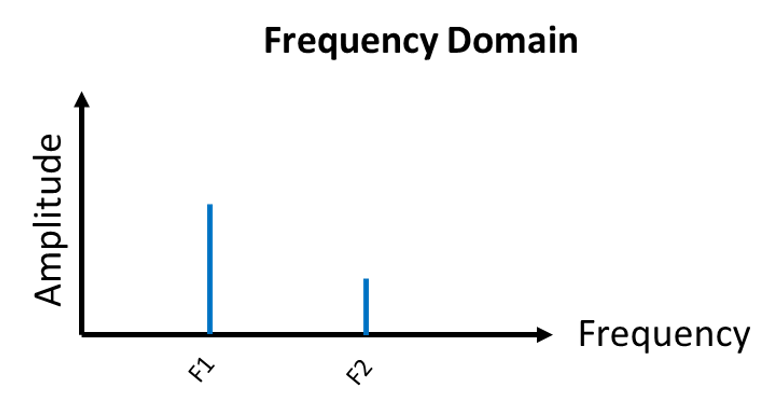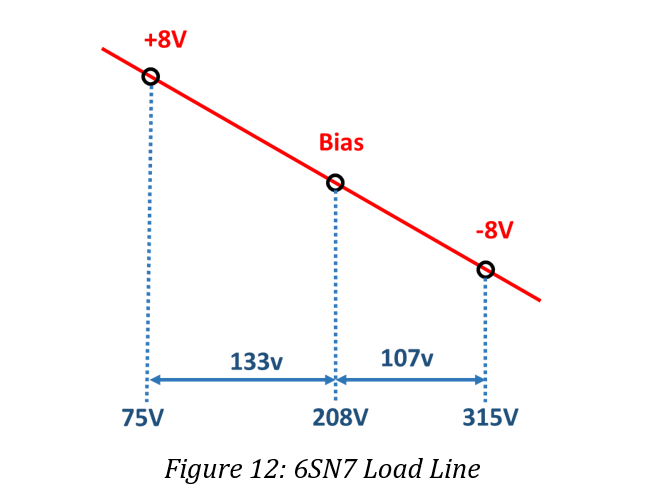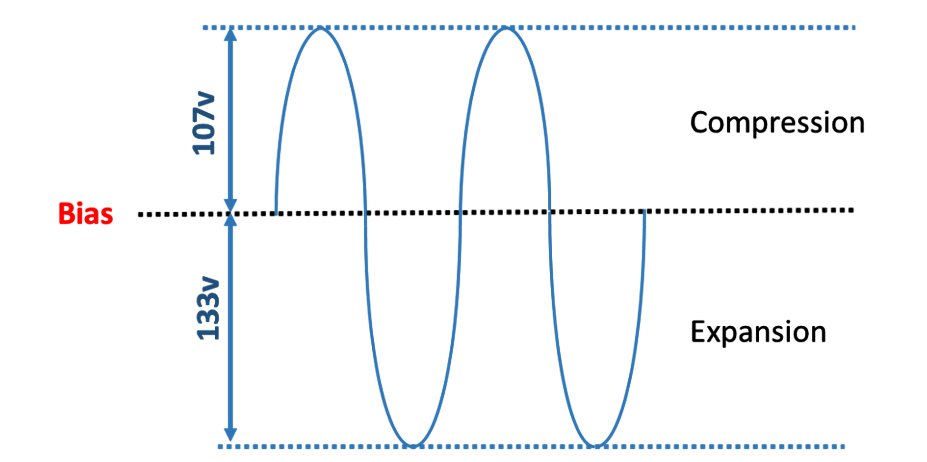top of page
Search

# Not all Distortion is Created Equal; A Guide to THD & THD+N

A Guide to THD and THD+N
.pdf

Distortion is arguably the most commonly compared specification between audio products. Consumers often use this as the first technical benchmark when purchasing a piece of equipment.

Even the name “distortion” has a bad connotation. Nobody wants distortion added to their audio, right?

This article is a discussion on what distortion (specifically THD) actually is, how it is measured, and how different amplifiers with the same distortion specification can actually sound very different.

1. Distortion

Distortion is a generic term used to describe unwanted artifacts added to an audio signal as it passes through an amplifier, preamplifier, ADC or DAC. Essentially any and every component a signal passes through (audio or other) adds some amount of distortion.

It is an inevitability of the world we live in. Nothing is perfect.

2. Thinking in Terms of Frequency

First, to understand THD we must think about audio signals in a different way.

We are all familiar with how signals look vs time. We can remember high school math functions like Sine, Cosine, and Tangent. With elapsed time, the amplitude varies positive and negative around a center point with a fixed amplitude and frequency. The X-axis is time, the Y-axis is the amplitude at any given point in time. This is known as the time domain since our perspective is relative to time.

Let’s now think of one of these signals displayed on a set of axes where the X-axis is the frequency of the signal, and the Y-axis is the amplitude. This is known as the frequency domain. Our view shows the spectral (frequency and amplitude) content of a signal.Figure 1: Time Domain Signal Plot

We can take the signal above and now view it in the frequency domain as a spectral plot.Figure 2: Frequency Domain Plot of the Signal Above

Note the Y-axis displays a vertical bar that reaches the same amplitude as before. The bar is located on the X-axis at a frequency corresponding to the frequency of the time domain signal.

Why is this useful?

Because signals are often much more complex than a simple single frequency sine wave. By using a spectral plot, we can transform a complex time domain signal and understand all the frequencies and the associated amplitude of each of those frequencies for additional analysis.

If we have a signal comprised of 2 frequencies and view the signal in the frequency domain, we can easily unravel the contents of the signal.Figure 3: Two Frequency SignalFigure 4: Two Frequency Signal Broken into its Components

To take this one step further, any time domain wave shape can be created though the addition of many pure tone waves of varying frequency and amplitude.

This also works in reverse. The most complex of waveforms can be represented in the frequency domain as many pure tone signals of different amplitudes.

This is called the Fourier Series.

Fourier, a French mathematician, proved this theory and provided the foundation for most signal processing techniques today.

The measurement of THD uses this concept.

3. THD - Total Harmonic Distortion

For audio equipment, THD is measured by injecting a known sinusoid signal into the Device Under Test (DUT) and comparing

the output of the device to the input signal.

Since the input signal is our reference, we can think of the distortion artifacts as harmonics (or frequency multiples) of the fundamental signal in the frequency domain.

These harmonics are then aggregated and compared to the input signal. Hence, the measurement we make is the total of all resulting harmonics or Total Harmonic Distortion.Equation 1: THD% Calculation

In Equation 1, the numerator is a power summation of the amplitudes of all the harmonics (second harmonic H2, third harmonic H3, fourth harmonic H4, etc). The denominator is the fundamental or input test signal amplitude. The fundamental is also referred to as the first harmonic but we will avoid using this terminology to prevent confusion. This gives us a ratio of the signal we care about (the fundamental) relative to all of the unwanted signals (the harmonics).

The source of these harmonics in audio equipment is due to the nonlinearities that exist in all circuit components as well as the overall circuit design and topology choices made… more on that later.

3.1 Measuring THD

Below shows the flow through of an audio device, where a sine wave fundamental signal is injected into the input of the DUT. The output is monitored by an audio analyzer which gives us the THD as a number.Figure 5: THD Test Signal Chain

If we look at the output from the DUT in the frequency domain, we may see something like the following where the DUT has added the harmonics H2, H3, H4 and H5.Figure 6: Fundamental and Harmonics

So how does an audio analyzer actually compute THD?

The audio analyzer duplicates the signal from the DUT on two separate paths.Figure 7: THD Audio Analyzer Diagram

Using specially designed filters in each path, path 1 effectively calculates the numerator and path 2 denominator of the THD calculation in Equation 1.

Path 1: The first path uses a filtering circuit to remove the fundamental tone from the rest of the signal. This type of filter is called a notch filter. It is highly tuned to only attenuate a very narrow frequency band. The notch filter is placed at the same frequency as the fundamental, effectively removing it from the signal. This passes the remaining harmonics downstream.Figure 8: Notch Filter Removes Fundamental

Path 2: The second path does the exact opposite as the first. It is a selective filter that is highly tuned to only pass a very narrow frequency band. This is called a band pass filter. It effectively removes all frequencies other than the fundamental.Figure 9: Bandpass Filter Leaves Fundamental

With each of these paths working in parallel, the output amplitudes of each path can be compared and computed into the THD of the DUT.

Note: It is worth noting here that even our fundamental source will have some amount of distortion. Again, this is unavoidable. The goal of any quality audio analyzer is to provide a fundamental test signal that has substantially lower distortion than the DUT itself. This will ensure that the DUT is being measured accurately.

A common check of any distortion measurement equipment is a loopback test where the DUT is removed and the fundamental signal source is fed directly into the analyzer.

This will determine the residual distortion of our measuring equipment.

4. THD+N - Total Harmonic Distortion Plus Noise

With a solid understanding on THD, we now must make a modification… We must account for noise.

Noise, like THD, is unavoidable. If you live on earth, then the physics of this planet dictate that all electrical signals will have noise. By considering noise of the DUT, we can reason that the signal amplitude is never truly zero between each harmonic in our spectral plots.

The noise floor of the DUT is fixed and contributes to the overall amplitude measured by audio analyzers.Figure 10: Noiseless vs Noisy DUT

In figure 10, notice how the additional of noise in the right plot sets the “floor” of our measurement. The amplitude never decays to zero. In this example, the noise masks the higher frequency H4 and H5 harmonics because their amplitude is lower than the noise floor.

Therefore THD+N is calculated with the addition of a noise term in the numerator. Noise is an additional unwanted artifact.Equation 2: THD+N% Calculation

In reality, this is how most audio analyzers actually measure distortion. The notch filter cannot remove the noise. Therefore, by default, the measurement made is almost always a THD+N value. Noise is a fact of life and part of the audio system.

4. Some manufacturers specify THD not THD+N

It is true that some manufacturers do specify THD rather than THD+N. If you don’t see the “+N” then noise is not part of the measurement!

While not technically incorrect, this is often done to mislead customers. For those who haven’t read this article (lucky you), many assume THD and THD+N are the same or don’t even notice the difference in the tiny print when comparing between products. Because THD+N includes noise, THD+N will always result in a higher distortion figure than just THD.

Most of if not all audio analyzers, including the gold standard Audio Precision, measure THD+N.

To remove the unavoidable effects of noise, THD is usually computed manually. Typically, a frequency domain spectral analysis of the DUT is taken and the peak amplitude of each harmonic is recorded and then computed by hand using Equation 1.

5. Sources of THD+N

As discussed, noise is one of the contributing factors to the total THD+N. Higher noise means higher THD+N. Any artifact not associated with the fundamental test signal, even harmonics of the power line rectification (60Hz, 120Hz, 240Hz etc.) are part of the combined noise of an audio system.

Harmonic distortion products are those which are related to the fundamental and occur as multiples of the fundamental frequency. For example, using a 1KHz fundamental test tone, the first distortion product we would expect to see is 2KHz which is the second harmonic of the fundamental. The third harmonic would be 3KHz and so on.

The generation of harmonics from a given fundamental input tone is due to non-linearity in the amplifier. The input never appears perfectly at the output of any system. The primary cause of nonlinearities are the amplifying devices themselves, tubes or transistors.

When an amplification device like a vacuum tube operates, its operating conditions setup a load profile over the tubes nominal operating characteristics. This is called a load line.

Let’s build a Class-A amplifier with a 6SN7 triode tube. If we operate the tube with a 350V supply and a 35KΩ resistor between the supply and the tube plate, we get a load line defined by this resistor value and the supply voltage.

Now let’s set up the nominal operating point (known as setting the bias) for this tube at -8V. Since audio signals are alternating polarity, we can handle up to a +8V peak signal amplitude for a maximum input signal of 0V on the tube grid. Conversely, we can handle -8V negative signal amplitude for minimum input signal of -16V on the tube grid.If we remove tube curves of the 6SN7 tube and look at the line drawn, we can see where linearity comes into play.Figure 12: Drawn 6SN7 Load Line

Notice how the tube output voltage on the X-axis is not linear relative to our bias point.

208V - 75V = 133V

315V – 208V = 107V

Although the input single swing is linear (+8 and -8V) the output voltage is not! We have a 20% difference in the voltage across the tube for the same input signal swing!

We can use this load line to draw the shape of a signal passing though this stage.Figure 13: Signal Shape from Load Line

On the -8V side of the bias point, the output signal is compressed. On the +8V side of bias point, the output signal is expanded. An ideal amplifying device would amplify the signal by the same amount for a given input signal amplitude.

If we follow this signal for a full cycle starting at the peak of the compression side, the signal continually expands and then compresses within a full cycle of our input. This creates a second harmonic, or small doubling frequency effect relative to the input signal due to the asymmetrical waveform.

Our simple 6SN7 Class-A amplifier generates harmonics and thus THD!

6. Not all Distortion is Created Equal

THD+N is useful for benchmarking a product, but it doesn’t tell the full story.

As discussed, THD+N is a combined value of all unwanted harmonics and noise. However, it says nothing about how the harmonics are related in frequency.

6.1 Even Order Distortion

In the previous example, we saw how a sample Class-A amplifier produces a small frequency doubling effect due to the 6SN7 tube characteristics. This is known as even order distortion. Regardless of the fundamental frequency, the output harmonics are an even multiple of the input signal frequency.

For example, 1KHz input signal will produce 2KHz, 4KHz, 6Khz, 8KHz and so on. The harmonic train will continue until the amplitude of the harmonics falls below the noise floor of the amplifier and are no longer discernable.

Since even order distortion is always an even multiple of the input signal, the harmonics are always “in tune”. Think of this as octaves on a piano. Octaves are the double of the initial frequency. If we play middle A (440Hz) on a piano as our fundamental, we would find our harmonics at H2: A (880Hz), H4: A (1760Hz) and H6: E(2640Hz). These notes all go well together.

Although harmonic distortion is present and we can measure it on an audio analyzer, to our ears, it is not very offensive or even perceivable.Figure 14: Even Order Distortion Profile

Audio amplifiers with dominant even order distortion can often have higher levels of THD+N without listeners perceiving any distortion.

This is the approach often used in Class-A single ended triode (SET) tube amplifiers. The circuit is optimized to be as simple as possible, providing excellent sonic results with zero negative feedback. Building a complex circuit to drive down THD isn’t usually necessary if designed properly.

6.2 Odd Order Distortion

Odd order distortion is not an even multiple of the input frequency but rather an odd multiple. No more doubling here!Figure 15: Odd Order Distortion Profile

To use our piano analogy, odd order harmonics would be like playing middle A (440Hz) as our fundamental and finding our harmonics falling at H3: E (1320Hz), H5: C# (2200Hz), and H7: somewhere between F#-G (3080Hz).

This more complex series can alter the sound of music and create dissonant sounds not musically associated with the input signal. This creates sounds which clash and compete with each other if the level or distortion is not kept in check.

What sort of audio equipment creates odd order distortion?

Any differential amplifier by nature (for example a differential XLR input on a preamp, or a push-pull output amp), will create a dominant odd order harmonic profile.

If we take our simple 6SN7 Class-A amplifier from before and duplicate it such that we have 2 inputs and 2 outputs that are operating out of phase (a basic differential amplifier), then the output signal will have compression on both the positive and negative signal extremes. If we drive the amplifier very hard into clipping, we will get a response that looks like a square wave, flat peaks at the maximum and minimum voltage swing.

Our friend Fourier proved that a square wave can made by summing pure sinusoidal tones at odd harmonics. Therefore, any symmetrical distortion waveform is full of odd order harmonics.

Furthermore, the differential nature of a differential amplifier tends to cancel even harmonics reducing the ratio of even order to odd order harmonics even further.

7. A Few Closing Notes

Any amplifier design will have some amount of even and odd order harmonics. The examples given above discuss why specific amplifier topologies and designs tend to be dominant in even or odd order distortion, but they are not excusive to one or the other.

Also, while not widely specified, the bandwidth used to measure the harmonics and noise to arrive at a THD+N number matters. If we measure THD+N over a 100KHz bandwidth, a higher THD+N number will be measured since more noise and harmonics will fit in a 100KHz frequency window compared to a strict audio window of 20KHz.

In fact, most Class-D amplifiers are measured with a very sharp AES-17 filter placed at 20KHz. This is designed to remove the PWM switching frequency (typically 300-500KHz) from the audio output. Without this filter, the PWM switching could be measured by an audio analyzer as part of the harmonic spectrum.

With this type of filter, it is not uncommon to see the THD+N drop dramatically to very low values at 10KHz. Why?

The 2nd harmonic of 10KHz is 20Khz which is being filtered out of the measurement! If the measurement bandwidth was increased, then no drop would be present, however as mentioned before, the THD+N measured everywhere else would be higher too!

bottom of page